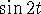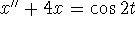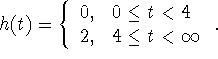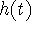# MA2051 - Ordinary Differential EquationsSample Exam 1 - B96

### Second Exam - Originally Given 1993 A Term

Covers 5.1, 5.3, 6.1-6.6, 7.1, 7.2, 8.1-8.3, 11.1-11.4.

Instructions: Do your work on the paper provided. Put your name and section number on that paper. Work neatly. Show your work. JUSTIFY YOUR ANSWERS.

1 35 pts
When a mass of 200 gm is added to a spring, it deflects 8 cm.

A
Suppose a mass of 200 gm is suspended from this spring and that the mass is started in motion from its equilibrium position with a downward push of 6 cm/s. Write a model for the position of the mass. (You don't need to derive the model from scratch. Use the usual coordinate system: up is positive, and the origin is the rest position of the mass.)

B
Find an expression for the position of the mass as a function of time by solving the initial-value problem you wrote in part (a).

C
Find the period of oscillation of the mass.

D
Find the maximum displacement of the mass.

2 20 pts
Note that solutions of x'' + 4x = 0 are,.

A
Find a particular solution of.

B
Find a general solution of this equation.

C
If this equation were a model of a spring-mass system, would you conclude that the displacement of the mass is bounded or unbounded? Give a physical reason for the behavior you observe.

3 25 pts
Consider the system

y' = -y + 3z
z' = 3y - z.

A
Find the characteristic values of this system.

B
Find a general solution of this system.

C
Find a solution that satisfies the initial conditions y(0) = 0, z(0) = 4.

#### This Topic will not be covered on the Exam

4 20 pts
DefineA
Find.

B
Find the Laplace transform of the solution of the initial-value problem y' - y = h(t), y(0) = 4.

C
Find the solution of this initial-value problem.

```

© 1996 by Will Brother.
All rights Reserved. File last modified on December 6, 1996.

```